# Estimating Ending Inventory

It is very time-consuming for a company to physically count the units of goods in its inventory. In fact, some companies shut down their operations near the end of their accounting year just to perform inventory counts. Often a company assigns one set of employees to count and tag the items and another set to verify the counts. If a company has outside auditors, they will be there to observe the process. (Even if the company's computers keep track of inventory, the computer quantities must be verified by physically counting the goods at least once per year.)

If a company using the periodic inventory system counts its inventory only once per year, it must estimate its inventory at the end of each month in order to prepare meaningful monthly financial statements. In fact, a company may need to estimate its inventory for other reasons as well. For example, if a company suffers a loss due to a disaster such as a tornado or a fire, it will need to file a claim for the approximate cost of the inventory that was lost. (An insurance adjuster will also compute the amount independently so that the company is not paid too much or too little for its loss.)

## Estimating Inventory: Gross Profit Method

The gross profit method for estimating the cost of the ending inventory uses information from a previously issued income statement. To illustrate the gross profit method we will assume that ABC Company needs to estimate the cost of its ending inventory on June 30, 2022.

ABC's latest income statement (which is representative of current conditions) contained the following information: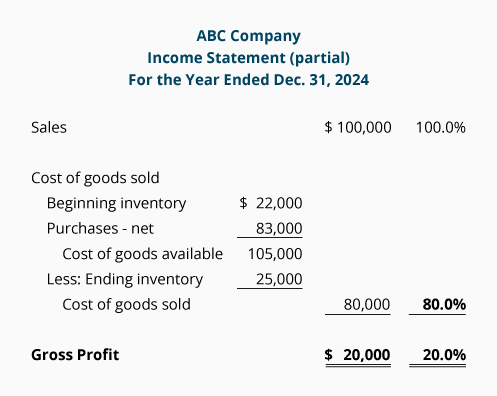From ABC's information we see that the company's gross profit is 20% of sales, and that the cost of goods sold is 80% of sales. If those percentages are reasonable for the current year, we can use them to estimate the cost of the inventory on hand as of June 30, 2022.

While an algebraic equation could be used, we prefer to simply use the income statement format. We will prepare a partial income statement for the period beginning after the date when inventory was last physically counted, and ending with the date for which we need the estimated inventory cost. In this case, the income statement we prepare will be from January 1, 2022 until June 30, 2022.

Some of the amounts needed can be obtained from sales records, customers, suppliers, earlier financial statements, etc. For example, sales for the first half of the year 2022 are taken from the company's records. The beginning inventory amount is the ending inventory reported on the December 31, 2021 balance sheet. The purchases information for the first half of 2022 is available from the company's records or its suppliers. The amounts that are available are shown in italics in the following partial income statement: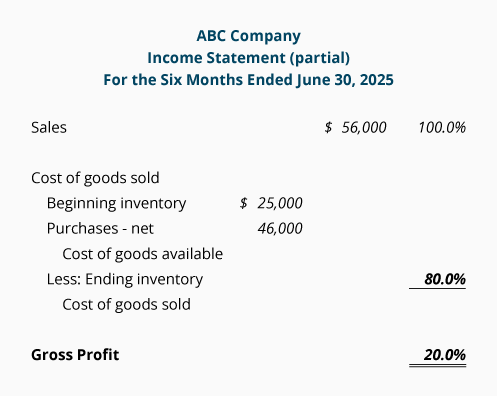We will fill in the rest of the statement with the answers from the following calculations. The calculation amounts in italics come from the statement above. The calculation amounts in bold will be used to complete the above section of the income statement: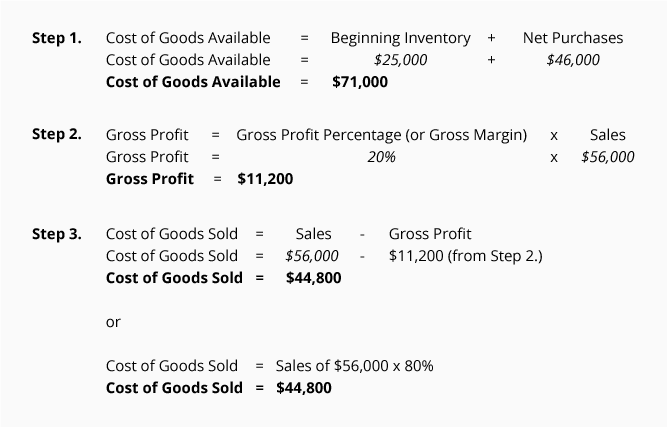Inserting this information into the income statement yields the following: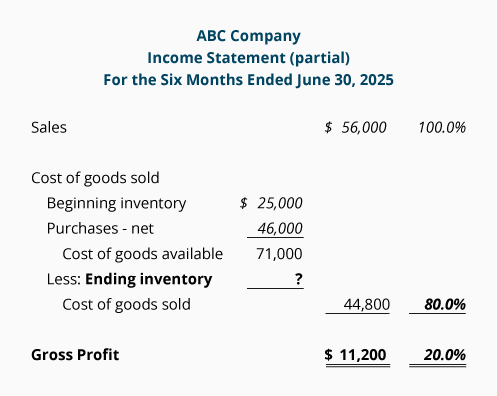Next, we need to compute the ending inventory amount. This is done by subtracting the cost of goods sold from the cost of goods available as shown here: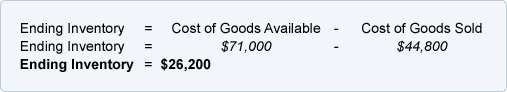Below is the completed partial income statement with the estimated amount of ending inventory at \$26,200. (Note: Always recheck the math on the income statement to be certain you computed the amounts correctly.)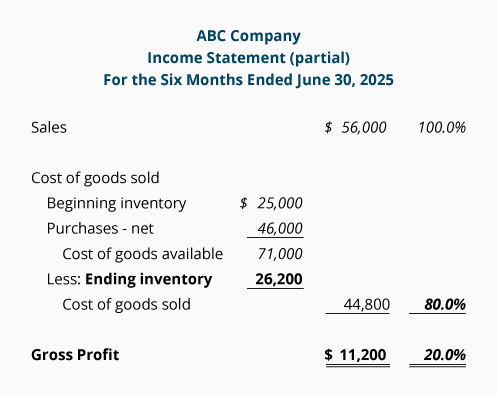## Estimating Inventory: Retail Method

Another method for estimating inventory is the retail method. This method can be used by retailers who have their merchandise records in both cost and retail selling prices. A very simple illustration of using the retail method for estimating the cost of ending inventory (using hypothetical amounts unrelated to earlier examples) is shown here: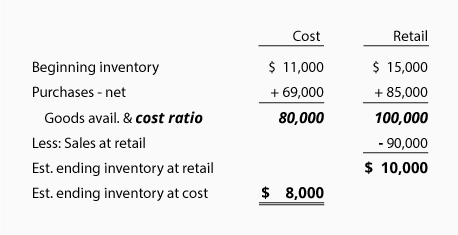Notice that the cost amounts are presented in one column and the retail amounts are listed in a separate column. The Goods Available amounts are used to compute the cost-to-retail ratio. In this case the cost of goods available of \$80,000 is divided by the retail amount of goods available of \$100,000. Therefore, the cost-to-retail ratio, or cost ratio, is 80%. The estimated ending inventory at cost is the estimated ending inventory at retail of \$10,000 times the cost ratio of 80% equals \$8,000.

#### Take Our Practice Quiz

We recommend that you now take our free Practice Quiz for this topic so that you can...

• See what you know
• See what you don't know